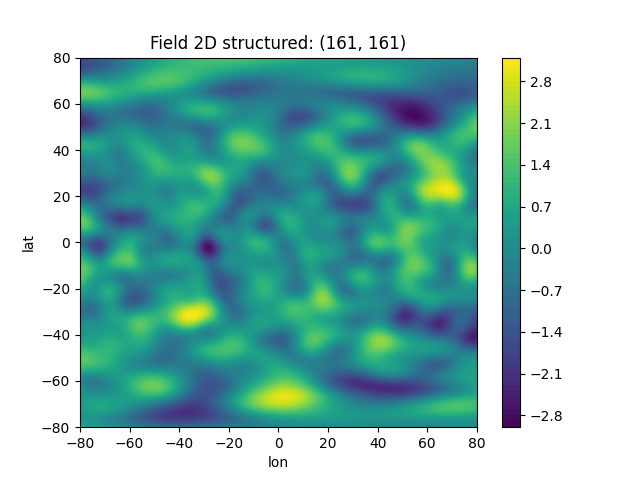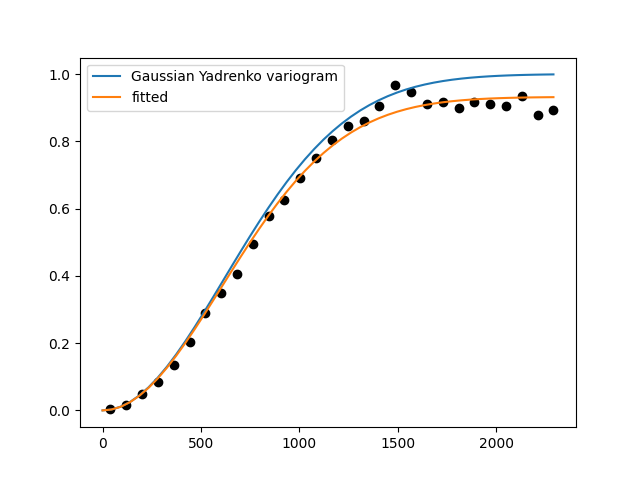# Working with lat-lon random fields

In this example, we demonstrate how to generate a random field on geographical coordinates.

First we setup a model, with latlon=True, to get the associated Yadrenko model.

In addition, we will use the earth radius provided by EARTH_RADIUS, to have a meaningful length scale in km.

To generate the field, we simply pass (lat, lon) as the position tuple to the SRF class.

import gstools as gs

model = gs.Gaussian(latlon=True, var=1, len_scale=777, rescale=gs.EARTH_RADIUS)

lat = lon = range(-80, 81)
srf = gs.SRF(model, seed=1234)
field = srf.structured((lat, lon))
srf.plot()This was easy as always! Now we can use this field to estimate the empirical variogram in order to prove, that the generated field has the correct geo-statistical properties. The vario_estimate routine also provides a latlon switch to indicate, that the given field is defined on geographical variables.

As we will see, everthing went well… phew!

bin_edges = [0.01 * i for i in range(30)]
bin_center, emp_vario = gs.vario_estimate(
(lat, lon),
field,
bin_edges,
latlon=True,
mesh_type="structured",
sampling_size=2000,
sampling_seed=12345,
)

ax = model.plot("vario_yadrenko", x_max=0.3)
model.fit_variogram(bin_center, emp_vario, nugget=False)
model.plot("vario_yadrenko", ax=ax, label="fitted", x_max=0.3)
ax.scatter(bin_center, emp_vario, color="k")
print(model)Gaussian(latlon=True, var=1.02, len_scale=8.3e+02, nugget=0.0, rescale=6.37e+03)


Note

Note, that the estimated variogram coincides with the yadrenko variogram, which means it depends on the great-circle distance given in radians.

Keep that in mind when defining bins: The range is at most, which corresponds to the half globe.

Total running time of the script: ( 0 minutes 10.081 seconds)

Gallery generated by Sphinx-Gallery